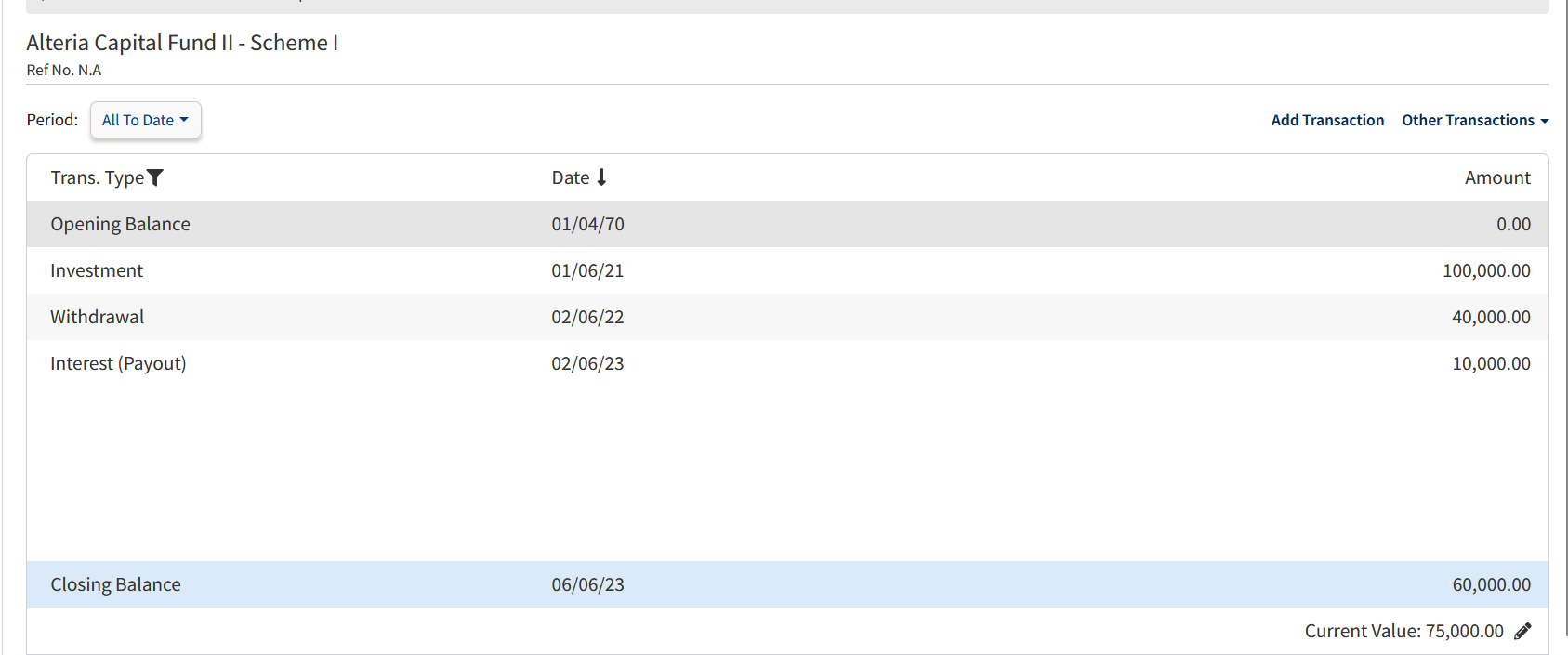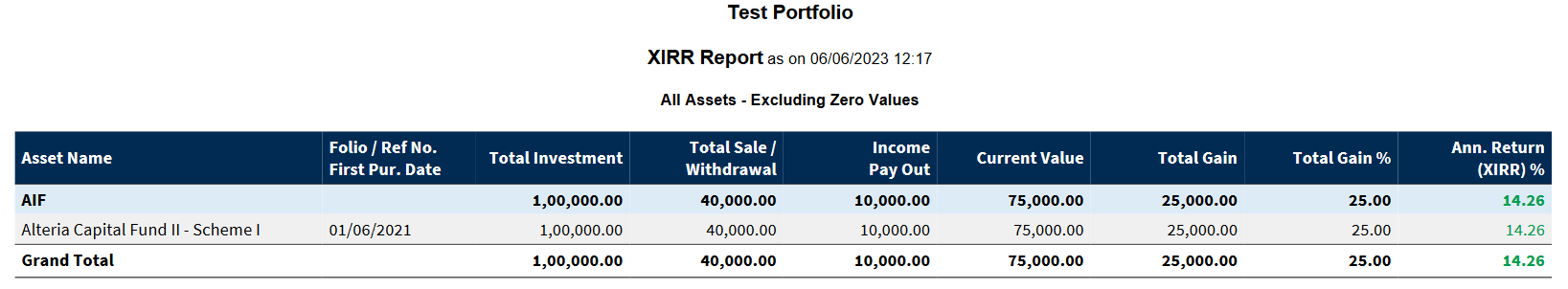# How MProfit computes Total Gain and % Total Gain in XIRR reports?

Modified on Tue, 06 Jun 2023 at 12:32 PM

Consider this one example to understand how the total gain value and % gain is calculated in MProfit.

AIF Asset - Alteria Capital Fund II - Scheme I

Total Investment - 100000
Withdrawal/Sell - 40000
Interest/Income Payout - 10000
Current Value - 75000

Screenshot of transaction view for your reference:Here Total Gain = (Current value - Total Investment) = 1,00,000 - 75,000 = Rs.25,000.

Total Gain in % = (100 * Total Gain / Total Investment) = 100 * 25,000 / 1,00,000 = 25%

Screenshot of XIRR Report showing Total Gain and Gain in % values.